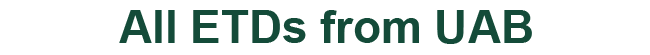### Multiplicities of Polygon Numbers

John C Mayer

Marius Nkashama

Frank Patane

Mahmut Unan

Thesis

2022

#### Degree Name by School

Master of Science (MS) College of Arts and Sciences

#### Abstract

Imagine that we have an unlimited number of congruent equilateral triangles. We define a trapezoid number as the number of equilateral triangles used to tile a trapezoid. Similarly, we define a parallelogram number as the number of equilateral triangles needed to tile a parallelogram. We define the multiplicity of a trapezoid or parallelogram number as the number of ways we can construct the polygon for given number up to congruence. We show a parallelogram number can always be written as 2kh and a trapezoid number as h(2k + h), where we define the height h as the length of the non-parallel sides, and the width k as the length of the shorter of the parallel sides, all in unit triangle lengths. The relationship between multiplicities and trapezoid/parallelogram numbers can be defined through equations relating the multiplicity to the number’s prime factorization. Our findings extend to multiplicities of hexagon numbers, or the number of congruent equilateral triangles tiling an equiangular hexagon. Based on numerical evidence, Hale et. al.  conjecture that “the multiplicity of a hexagon number is a function of its prime factorization.” They showed that if the height p of a hexagon is a prime number congruent to 1 (mod 3), then it produces all residues (mod 2p). Our main result is: If the height of a hexagon is pq, where p > 5 is the product of prime factors congruent to 1 (mod 4), and q is the product of unique prime factors congruent to 3 (mod 4), then the height pq produces all residues (mod 2pq). iii

COinS